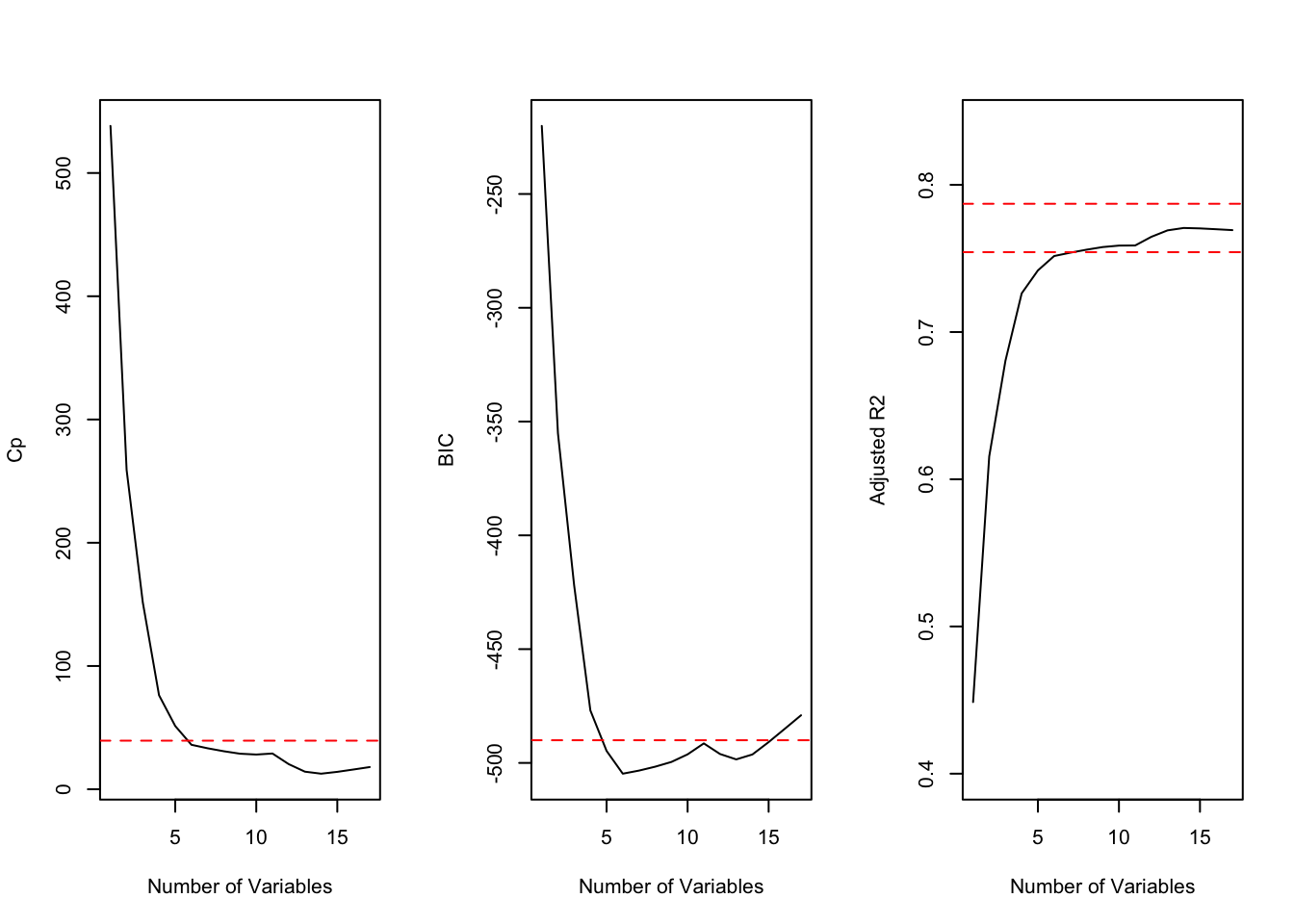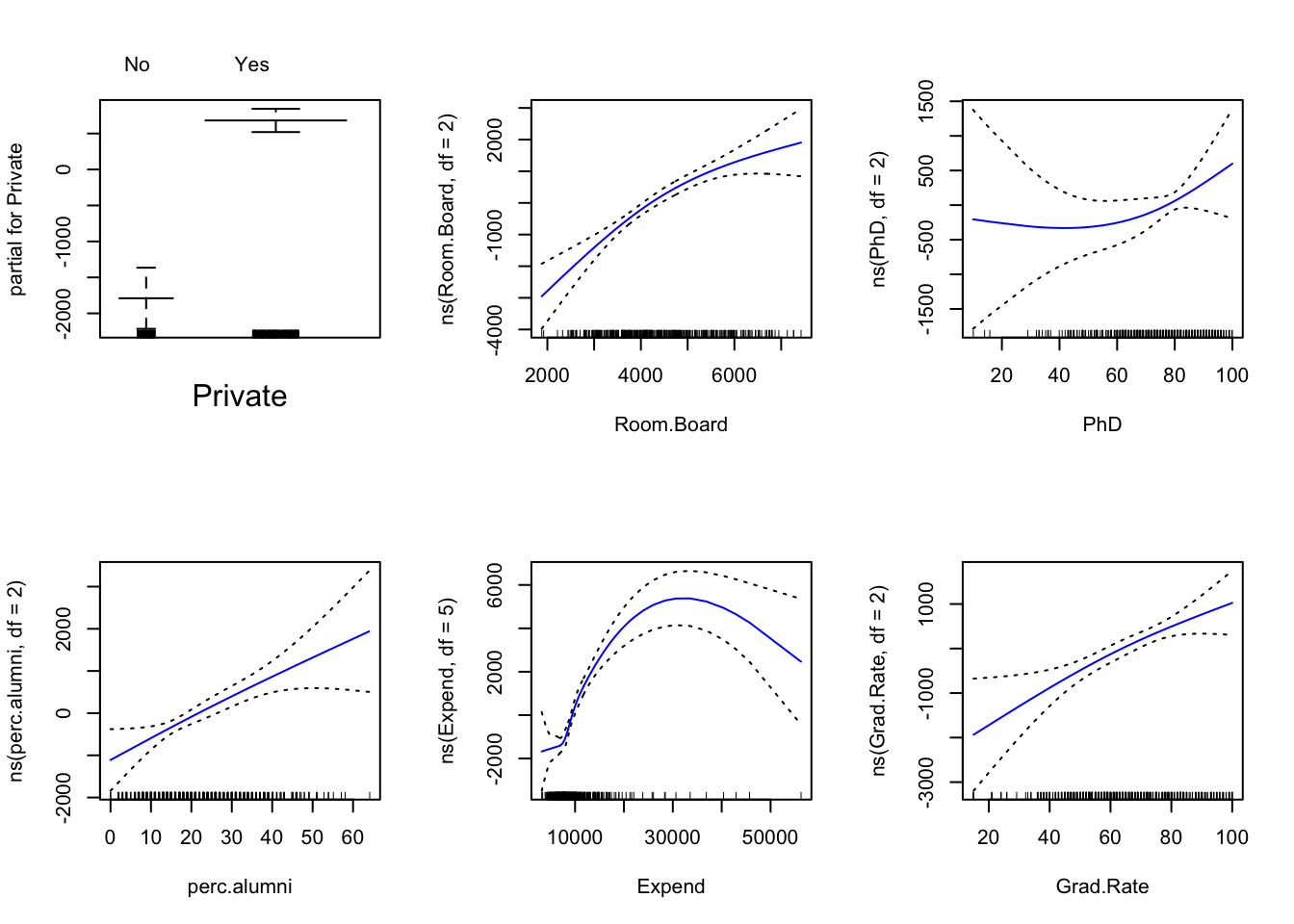### Exercise 7.1

This question relates to the College dataset from the ISLR package.

1. Split the data into a training set and a test set. Using out-of-state tuition as the response and the other variables as the predictors, perform appropriate model selection of your choice (from day6) on the training set in order to identify a satisfactory model that uses just a subset of the predictors.
library(ISLR)
library(glmnet)
## Loading required package: Matrix
## Loading required package: foreach
## Loaded glmnet 2.0-18
library(leaps)
set.seed(11)
sum(is.na(College))
##  0
train.size <-  nrow(College) / 2
train <-  sample(1:nrow(College), train.size)
test <-  -train
College.train <-  College[train, ]
College.test <-  College[test, ]
train.mat <-  model.matrix(Outstate ~ . , data = College.train)
test.mat <-  model.matrix(Outstate ~ . , data = College.test)
grid <-  10 ^ seq(4, -2, length = 100)
mod.lasso <-  cv.glmnet(train.mat, College.train[, "Outstate"], alpha = 1,
lambda = grid, thresh = 1e-12)

lambda.best <-  mod.lasso$lambda.min lambda.best ##  65.79332 lasso <- glmnet(train.mat, College.train[, "Outstate"], alpha = 1, lambda = grid) lasso.coef <- predict(lasso, type= "coefficients", s = lambda.best) lasso.coef ## 19 x 1 sparse Matrix of class "dgCMatrix" ## 1 ## (Intercept) -1.715631e+03 ## (Intercept) . ## PrivateYes 2.196976e+03 ## Apps . ## Accept 5.196523e-02 ## Enroll . ## Top10perc . ## Top25perc . ## F.Undergrad . ## P.Undergrad . ## Room.Board 9.199431e-01 ## Books . ## Personal -2.929415e-01 ## PhD 5.158463e+00 ## Terminal 2.508018e+01 ## S.F.Ratio -4.883095e+01 ## perc.alumni 5.678172e+01 ## Expend 2.006555e-01 ## Grad.Rate 2.798799e+01 LASSO hasn’t dropped any variables from our model, so it was a very successful attempt at model selection. Other approaches have been covered in the chapter (although not in the lecture). Best subset selection is a brute force approach but may be useful if we are determined in reducing the size of our model. set.seed(1) train <- sample(1:nrow(College), nrow(College)/2) test <- -train College.train <- College[train, ] College.test <- College[test, ] reg.fit <- regsubsets(Outstate~., data=College.train, nvmax=17, method="forward") reg.summary <- summary(reg.fit) par(mfrow=c(1, 3)) plot(reg.summary$cp,xlab="Number of Variables",ylab="Cp",type='l')
min.cp <-  min(reg.summary$cp) std.cp <- sd(reg.summary$cp)
abline(h=min.cp+0.2*std.cp, col="red", lty=2)
abline(h=min.cp-0.2*std.cp, col="red", lty=2)
plot(reg.summary$bic,xlab="Number of Variables",ylab="BIC",type='l') min.bic <- min(reg.summary$bic)
std.bic <-  sd(reg.summary$bic) abline(h=min.bic+0.2*std.bic, col="red", lty=2) abline(h=min.bic-0.2*std.bic, col="red", lty=2) plot(reg.summary$adjr2,xlab="Number of Variables",
ylab="Adjusted R2",type='l', ylim=c(0.4, 0.84))
max.adjr2 <-  max(reg.summary$adjr2) std.adjr2 <- sd(reg.summary$adjr2)
abline(h=max.adjr2+0.2*std.adjr2, col="red", lty=2)
abline(h=max.adjr2-0.2*std.adjr2, col="red", lty=2)BIC scores show 6 as the optimal size. Cp, BIC and adjr2 show that size 6 is the minimum size for the subset for which the scores are withing 0.2 standard deviations of optimum. We pick 6 as the best subset size and find best 6 variables using entire data.

reg.fit <-  regsubsets(Outstate ~ . , data=College, method="forward")
coefi <-  coef(reg.fit, id=6)
names(coefi)
##  "(Intercept)" "PrivateYes"  "Room.Board"  "PhD"         "perc.alumni"
##  "Expend"      "Grad.Rate"
1. Fit a GAM on the training data, using out-of-state tuition as the response and the features selected in the previous step as the predictors. Plot the results, and explain your findings.
library(gam)
## Loading required package: splines
## Loaded gam 1.16.1
gam.fit <-  gam(Outstate ~ Private + ns(Room.Board, df=2) +
ns(PhD, df=2) + ns(perc.alumni, df=2) +
ns(Expend, df=5) + ns(Grad.Rate, df=2),
data=College.train)
## Warning in model.matrix.default(mt, mf, contrasts): non-list contrasts
## argument ignored
par(mfrow=c(2, 3))
plot(gam.fit, se=TRUE, col="blue")** We discussed this type of graphs in the lecture. The fitted natural splines with +/- 2*SE confidence interval. Ticks at the bottom show density of the data (aka rug plot’).**

1. Evaluate the model obtained on the test set, and explain the results obtained.
gam.pred <-  predict(gam.fit, College.test)
gam.err <-  mean((College.test$Outstate - gam.pred)^2) gam.err ##  3415951 gam.tss <- mean((College.test$Outstate - mean(College.test$Outstate))^2) test.rss <- 1 - gam.err / gam.tss test.rss ##  0.7613443 We obtain a test RSS of 0.76 using GAM with 6 predictors. This is a slight improvement over a test RSS of 0.74 obtained using OLS. 1. For which variables, if any, is there evidence of a non-linear relationship with the response? summary(gam.fit) ## ## Call: gam(formula = Outstate ~ Private + ns(Room.Board, df = 2) + ns(PhD, ## df = 2) + ns(perc.alumni, df = 2) + ns(Expend, df = 5) + ## ns(Grad.Rate, df = 2), data = College.train) ## Deviance Residuals: ## Min 1Q Median 3Q Max ## -7151.7 -1109.0 -36.1 1305.9 7654.9 ## ## (Dispersion Parameter for gaussian family taken to be 3826578) ## ## Null Deviance: 6989966760 on 387 degrees of freedom ## Residual Deviance: 1427313633 on 373 degrees of freedom ## AIC: 6998.901 ## ## Number of Local Scoring Iterations: 2 ## ## Anova for Parametric Effects ## Df Sum Sq Mean Sq F value Pr(>F) ## Private 1 2022785948 2022785948 528.615 < 2.2e-16 *** ## ns(Room.Board, df = 2) 2 2003024353 1001512176 261.725 < 2.2e-16 *** ## ns(PhD, df = 2) 2 705105726 352552863 92.133 < 2.2e-16 *** ## ns(perc.alumni, df = 2) 2 326167935 163083967 42.619 < 2.2e-16 *** ## ns(Expend, df = 5) 5 426700704 85340141 22.302 < 2.2e-16 *** ## ns(Grad.Rate, df = 2) 2 78868463 39434231 10.305 4.403e-05 *** ## Residuals 373 1427313633 3826578 ## --- ## Signif. codes: 0 '***' 0.001 '**' 0.01 '*' 0.05 '.' 0.1 ' ' 1 Non-parametric Anova test shows a strong evidence of non-linear relationship between response and variables. ### Exercise 7.2 Apply bagging and random forests to a data set of your choice. Be sure to fit the models on a training set and to evaluate their performance on a test set. How accurate are the results compared to simple methods like linear or logistic regression? Which of these approaches yields the best performance? In this exercise we examine the Weekly stock market data from the ISLR package. set.seed(1) summary(Weekly) ## Year Lag1 Lag2 Lag3 ## Min. :1990 Min. :-18.1950 Min. :-18.1950 Min. :-18.1950 ## 1st Qu.:1995 1st Qu.: -1.1540 1st Qu.: -1.1540 1st Qu.: -1.1580 ## Median :2000 Median : 0.2410 Median : 0.2410 Median : 0.2410 ## Mean :2000 Mean : 0.1506 Mean : 0.1511 Mean : 0.1472 ## 3rd Qu.:2005 3rd Qu.: 1.4050 3rd Qu.: 1.4090 3rd Qu.: 1.4090 ## Max. :2010 Max. : 12.0260 Max. : 12.0260 Max. : 12.0260 ## Lag4 Lag5 Volume ## Min. :-18.1950 Min. :-18.1950 Min. :0.08747 ## 1st Qu.: -1.1580 1st Qu.: -1.1660 1st Qu.:0.33202 ## Median : 0.2380 Median : 0.2340 Median :1.00268 ## Mean : 0.1458 Mean : 0.1399 Mean :1.57462 ## 3rd Qu.: 1.4090 3rd Qu.: 1.4050 3rd Qu.:2.05373 ## Max. : 12.0260 Max. : 12.0260 Max. :9.32821 ## Today Direction ## Min. :-18.1950 Down:484 ## 1st Qu.: -1.1540 Up :605 ## Median : 0.2410 ## Mean : 0.1499 ## 3rd Qu.: 1.4050 ## Max. : 12.0260 train <- sample(nrow(Weekly), 2/3 * nrow(Weekly)) test <- -train Logistic regression glm.fit <- glm(Direction ~ . -Year-Today, data=Weekly[train,], family="binomial") glm.probs <- predict(glm.fit, newdata=Weekly[test, ], type = "response") glm.pred <- rep("Down", length(glm.probs)) glm.pred[glm.probs > 0.5] <- "Up" table(glm.pred, Weekly$Direction[test])
##
## glm.pred Down  Up
##     Down   28  33
##     Up    123 179
mean(glm.pred != Weekly$Direction[test]) ##  0.4297521 Bagging library(randomForest) ## randomForest 4.6-14 ## Type rfNews() to see new features/changes/bug fixes. Weekly <- Weekly[,!(names(Weekly) %in% c("BinomialDirection"))] bag.weekly <- randomForest(Direction~.-Year-Today, data=Weekly, subset=train, mtry=6) yhat.bag <- predict(bag.weekly, newdata=Weekly[test,]) table(yhat.bag, Weekly$Direction[test])
##
## yhat.bag Down  Up
##     Down   65  82
##     Up     86 130
mean(yhat.bag != Weekly$Direction[test]) ##  0.4628099 Random forests rf.weekly <- randomForest(Direction ~ . -Year-Today, data=Weekly, subset=train, mtry=2) yhat.bag <- predict(rf.weekly, newdata=Weekly[test,]) table(yhat.bag, Weekly$Direction[test])
##
## yhat.bag Down  Up
##     Down   59  73
##     Up     92 139
mean(yhat.bag != Weekly\$Direction[test])
##  0.4545455`

Best performance summary: Bagging resulted in the lowest validation set test error rate.